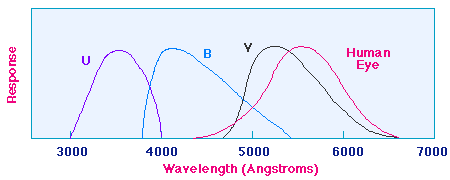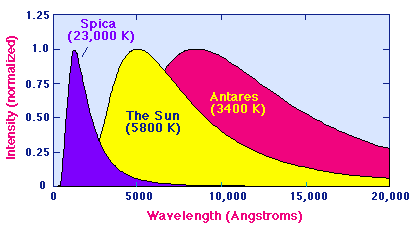Color Indices and Surface Temperatures of a Star

The section on Radiation Laws indicated that there is a relationship between the temperature of a blackbody and the location of the peak in the radiation distribution as a function of wavelength (Wien Law) . This allows definition of some continuous quantities called color indices that can be determined directly from observations and that are indirect indicators of temperature for a star.

### Astronomical Color Filters

Optical devices called filters may be devised that allow light to pass in a limited range of wavelengths. In astronomy, a variety of filters are used to emphasize light in a particular wavelength region, but the most common are called the U (ultraviolet), B (blue), and photovisual (V) filters. Their transmission of light as a function of wavelength, as well as the response of the average human eye, is illustrated in the following figure.Response of standard astronomical filters and the human eye

The names for the filters arise because the peaks for transmission for the U, B, and V filters are in the ultraviolet, blue, and yellow-green region of the spectrum (the human eye is most sensitive to the yellow-green region of the visible-light spectrum). Thus, astronomers can measure the intensity of light from a source like a star in each of these regions of the spectrum by passing the light collected by the telescope through the appropriate filter.

From the Planck Law radiation distributions illustrated below for several stars of differing surface temperatures, we see that generally the intensity of light from a star of given temperature will not be the same in the U, B, and V regions.(Note that for convenience in plotting these distributions have been normalized to unity at the respective peaks; by the Stefan-Boltzmann Law, the area under the peak for the hot star Spica is in reality 2094 times the area under the peak for the cool star Antares.)

### Color Indices

A color index is defined by taking the difference in magnitudes (which are related logarithmically to intensity) at two different wavelengths. Using the U, B, and V color filters, there are three independent possible such differences. For example, the B-V color index is defined by taking the difference between the magnitudes in the blue and visual regions of the spectrum and the U-B color index is the analogous difference between the UV and blue regions of the spectrum.

### Color Index Examples

The star Spica has apparent magnitudes U = -0.24, B = 0.7, and V = 0.9 in the UV, blue, and photovisual regions, respectively. The corresponding color indices are

B - V = 0.7 - 0.9 = - 0.2

U - B = -0.24 -0.7 = - 0.94

Generally, the negative values of these color indices are an indication that Spica is a hot star, with most of its radiation coming at shorter wavelengths. On the other hand, for Antares B = 2.7 and V=0.9, and the B - V color index is

B - V = 2.7 - 0.9 = 1.8

The positive value of B - V in this case is an indication that Antares is a cool star, with most of its radiation coming at longer wavelengths.